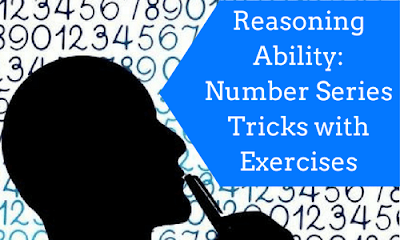New Student User - Use Code HELLO

# Reasoning Ability: Number Series Tricks## Number series

Number series is part in which many questions are asked in competitive exams. The number series will be asked in two ways
• Finding odd one out
• Find the missing number

In both the ways we have to find out that one particular number. To find that number we have to see the previous or next number pattern in that question and work on the same to get the required number. First we have to see the given number is prime, even, odd, perfect square, cube, particular multiply or division. In this article we are going to see some of the major patterns followed the number series questions with examples as follows

### Difference between numbers

In this we have to see the difference between the numbers

#### Example 1

11, 14, 19, 22, ?, 30
In the above: 11+3=14, 14+5=19, 19+3=22, 22+5=27, 27+3=30
So we have the answer by adding alternate numbers with 3 and 5 is 27

#### Example 2

4, 7, 12, ?, 28, 39
In the above: 4+3=7, 7+5=12, 12+7=19, 19+9=28, 28+11=39
So the answer by adding the series with the odd numbers 3,5,7,9,11 is 19

### Squares/cubes

In this we have to see the difference with respect to squares or cubes

#### Example 1

125,?,343,512,1331
In the above: 5³=125, 6³=216,7³=343,8³=512
So we have the answer by the cube of 6=216

#### Example 2

121,144,169,?,225
In the above series: 11²=121,12²=144,13²=169,14²=196,15²=225
So we have the answer by the square value of 14=196

### Prime numbers

In this we have to see the difference for having prime numbers

#### Example

2,3,?,7,11
In this series we see the order is prime number, so the missing number is 5

### Pattern change

In this pattern change we will see that in a single series two parts present

#### Example

2,9,?,1,8,4
In the above series 1st term 4th term is linked because 4th term ‘1’ is present and number next is ‘2’ which is present in 1st position2___1, 9___8, 5___4

### Odd man out series

In this we have to find the number which does not belong to that particular series

#### Example 1

2,4,6,8,10,11,12
In the above series 11 does not belong to that series as the series follow even number order

#### Example 2

3,5,7,12,17,19
In the above series follows prime number series but 12 is not a prime number
So the wrong number/ odd man out is 12

### Multiplication/division

In this we have to check the difference with respect to multiplication or division

#### Example 1

73205,6655,605,?,5
In this we can see that it is multiplication of 11

⇒5*11=55, 55*11=605, 605*11=6655, 6655*11=73205
So the answer for the series is 55

#### Example 2

40960,10240,2560,200,160,40
In this question we have to find the odd number or wrong number
The above series is divisible by 4
⇒40960÷4=10240,
⇒10240÷4=2560,
⇒2560÷4=640,
⇒640÷4=160,
⇒160÷4=40
So the answer is 200 as that number is the wrong and value that should come in its place is 640

### Multiple functions series

In this series will have many functions to solve the series to get the required answer

#### Example 1

11,24,50,?,206
In this series we need to multiply and add 11*2=22+2=24
⇒24*2=48+2=50
⇒50*2=100+2=102
⇒102*2=204+2=206
So the answer for the above series is 102

#### Example 2

10,26,74,?,650
In this series we have to multiply and subtract 10*3=30-4=26
⇒26*3=78-4=74
⇒74*3=222-4=218
⇒218*3=654-4=650
So the answer for the series is 218

## Exercises For Practice

### Exercise 1: Find the odd /wrong number:

1. 253,136,352,460,324,631,244
2. 52,51,48,43,34,27,16
3. 125,123,120,115,108,100,84
4. 4,6,8,9,10,11,12
5. 8,13,18,25,30,37,40
6. 8,29,125,343,1331
7. 2,5,10,17,26,37,50,64
8. 16,25,36,72,144,196,225
9. 46080,3840,384,48,24,2,1
10. 5,16,6,16,7,16,9,16,9.

### Exercise 2: Find the missing number:

1. 41,43,47,___,61,71
2. 4,5,7,10,14,___,25,32
3. 4,-8,16,-32,64,___
4. 4. 5,10,13,26,___,58,61,122
5. 8,7,11,12,14,17,17,22,___
6. 1,2,6,15,___,56
7. 1,3,10,21,64,129,___,777
8. 5,8,___,42,124,246,736
9. 6,12,48,___,384,768,3072
10. 32,36,___,61,86,122,171,235

### Exercise 1

1. Sum of the digits in each number except 324 is 10
2. Subtract 1,3,7,9,11, from successive numbers. So 34 is wrong
3. Prime numbers 2,3,5,7,11 from successively been subtracted so 100 is wrong
4. Each number is a composite number except 11.
5. The difference between two successive terms from the beginning are 7,5,7,5,7,5,. So 40 is wrong
6. Each number is cube except 29. So 29 is wrong
7. The pattern is x²+1, where x=1,2,3,4,5,6 etc, but 64 is wrong
8. Each number except 72 is a perfect square
9. The terms are successively divided by 12,10,8,6,… so 24 is wrong
10. Terms at odd places are 5,6,7,8,.. and even place is 16. So 9 is wrong

### Exercise 2

1. Each number is a prime number answer is 53
2. 2nd=(1st+1); 3rd=(2nd+2);4th=(3rd+3).. so answer is 19
3. Each number is the preceding number multiplied by -2. So 136 is answer
4. Numbers are alternatively multiplied by 2 and increased by 3. So answer is 29
5. There are two series (8,11,14,17,20)and (7,12,17,22) increasing by 3 and 5 respectively
7. A*2+1, B*3+1, C*2+1, D*3+1and so on. So the answer is 388
8. 8.2nd=(1st*2-2)=8
⇒3rd=(2nd*2-2)=22
⇒4th=(3rd*2-2)=42 and so on ,so the answer is 22
9. 2nd=(1st *2); 3rd=(2nd *4);4th=(3rd *2).. so answer is  96
10. 2nd=(1st +2²)=32+4=36
⇒ 3rd=(2nd+3²)=36+9=45
⇒ 4th=(3rd+4²)=45+16=61 and so on. So answer is 45.